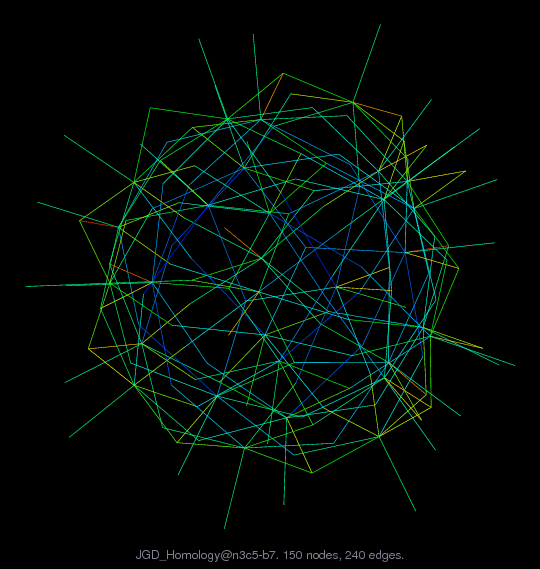Matrix: JGD_Homology/n3c5-b7

Description: Simplicial complexes from Homology from Volkmar Welker.(bipartite graph drawing)• Matrix group: JGD_Homology
• download as a MATLAB mat-file, file size: 1 KB. Use UFget(2095) or UFget('JGD_Homology/n3c5-b7') in MATLAB.

 Matrix properties number of rows 30 number of columns 120 nonzeros 240 structural full rank? yes structural rank 30 # of blocks from dmperm 1 # strongly connected comp. 1 explicit zero entries 0 nonzero pattern symmetry 0% numeric value symmetry 0% type integer structure rectangular Cholesky candidate? no positive definite? no

 author V. Welker editor J.-G. Dumas date 2008 kind combinatorial problem 2D/3D problem? no

Notes:

```Simplicial complexes from Homology from Volkmar Welker.
From Jean-Guillaume Dumas' Sparse Integer Matrix Collection,
http://ljk.imag.fr/membres/Jean-Guillaume.Dumas/simc.html

http://www.mathematik.uni-marburg.de/~welker/

Filename in JGD collection: Homology/n3c5.b7.30x120.sms
```

 Ordering statistics: result nnz(V) for QR, upper bound nnz(L) for LU, with COLAMD 1,738 nnz(R) for QR, upper bound nnz(U) for LU, with COLAMD 332

 SVD-based statistics: norm(A) 3.16228 min(svd(A)) 1.41421 cond(A) 2.23607 rank(A) 30 sprank(A)-rank(A) 0 null space dimension 0 full numerical rank? yes

 singular values (MAT file): click here SVD method used: s = svd (full (R)) ; where [~,R,E] = spqr (A') with droptol of zero status: ok